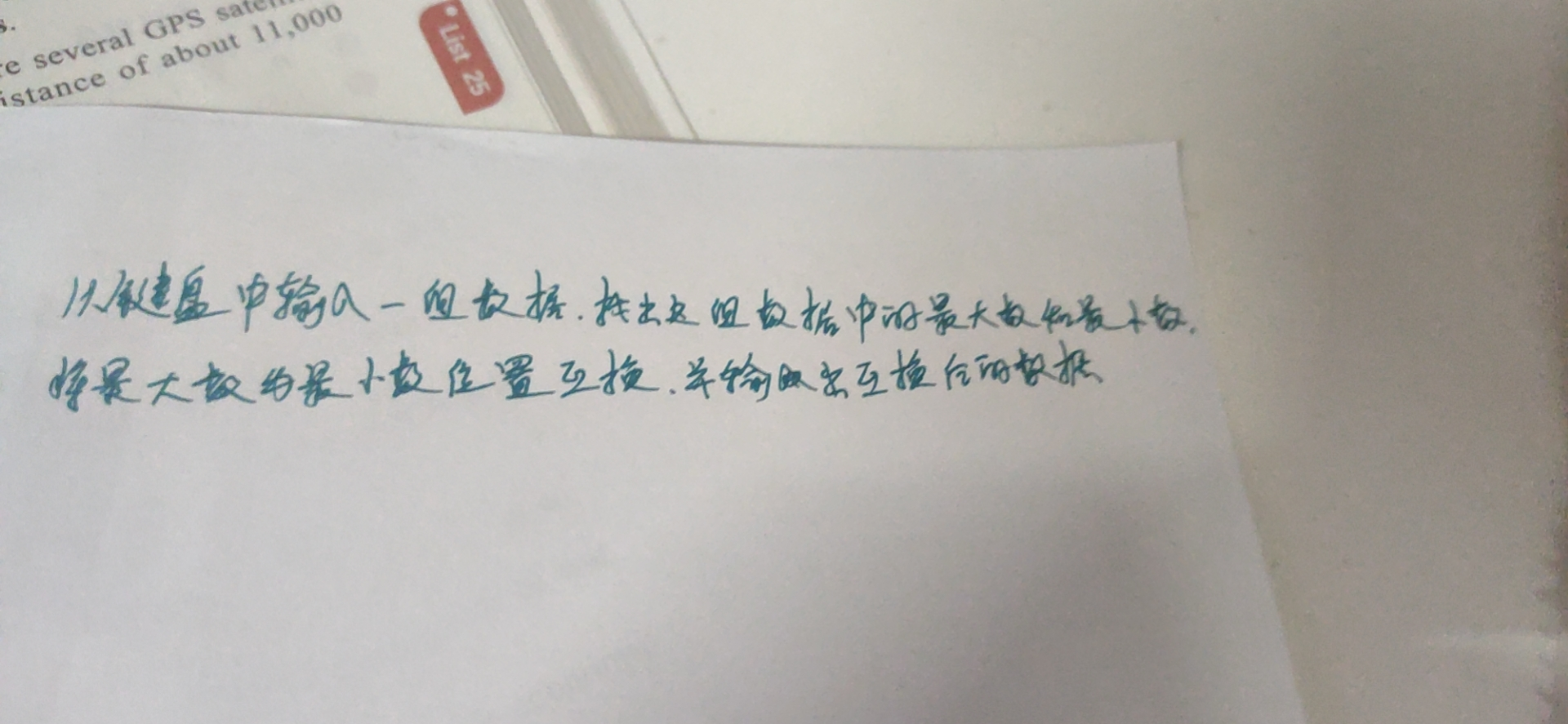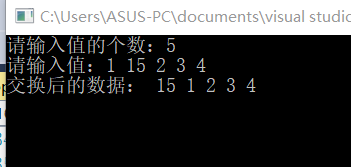2021-06-27 21:26

# 输入一组数据，将最大数与最小数互换，并输出互换后的数• 写回答
• 关注问题
• 收藏
• 邀请回答

#### 1条回答默认 最新

•登楼流樱 2021-06-27 21:41
已采纳
``````#include <stdio.h>

void main()
{
int arr, num, maxIndex=0, minIndex=0;
printf("请输入值的个数：");
scanf_s("%d", &num);
printf("请输入值：");
for (int i = 0; i < num; i++)
scanf_s("%d", &arr[i]);
int max = arr, min = arr;
for (int i = 0; i < num; i++)
{
if (max < arr[i])
{
max = arr[i];
maxIndex = i;
}
if (min > arr[i])
{
min = arr[i];
minIndex = i;
}
}
arr[maxIndex] = min;
arr[minIndex] = max;

printf("交换后的数据：");
for (int i = 0; i < num; i++)
printf(" %d", arr[i]);
getchar();
getchar();
}``````已采纳该答案
打赏 评论